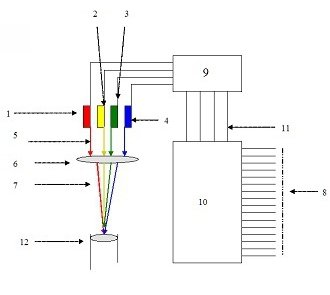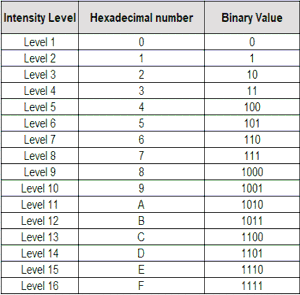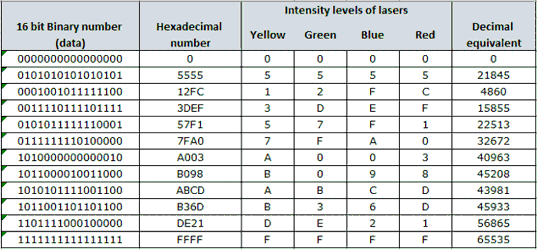# Optical Transceiver to Transmit Data through Multiple Lasers of variable intensities

Transfer 2 bytes single clock pulse through optical fibre
Patent application no. -  634/MUM/2001

Data transmission through variable intensity monochromatic lasers

The transceiver consist multiple monochromatic lasers and each laser has variable light intensities. Intensity of laser is controlled by voltage input to the source of laser. When the source emits laser at specific intensity level, the corresponding hexadecimal number assigned to that intensity level is transmitted. Four bit binary data is transmitted by one laser since each hexadecimal number is equivalent to 4 bit binary number.

A single variable intensity monochromatic laser transmits a hexadecimal number at every clock pulse.
The optical transmitter consists of four variable intensity monochromatic lasers. Each laser is of specific wavelength and no two lasers are of same wavelength. The intensity of each laser is controlled by voltage input to the source of that laser. Four lasers with 16 intensity levels each make 164 = 65536 combinations of different intensities and wavelengths.

These combinations are sufficient to transmit 16-bit binary number at one instance.

Invention (What is New)

Variable intensity laser: – The intensity of laser is controlled by voltage input to the source that emits laser. Laser source emit light at any of 16 different levels of intensities. The first level of intensity is LEVEL 1, this state occurs when laser is off; the sixteenth level of intensity occurs when laser is ON and emits light with maximum intensity. 14 intermediate levels of intensities are between Level 1 and Level 16. These 16 levels are evenly spaced (the difference between intensities of two consecutive intensity levels is equal). A hexadecimal number is assigned to each intensity level.

Diagram & DescriptionIn above diagram, 8 is input 16 bit number to be transmitted fed to processing circuit 10. This sends data to 9, which controls input voltages to individual lasers depending upon input number. The lasers are then collimated in to single beam using optical lenses and transmitted through optical fibre
The transmitter consists of four lasers. Each laser is of specific wavelength. The wavelengths (colors) (For Example RED, YELLOW, GREEN and BLUE). All four lasers are variable intensity lasers. The intensities of these lasers are controlled by voltage input to laser sources. Each source has an independent voltage input. The voltage input is provided by an input voltage controller circuit. The circuit generates separate voltage input for each laser depending upon hexadecimal number to be transmitted.

Sixteen bit binary data is converted into equivalent 4-digit hexadecimal number. Most significant digit of hexadecimal number controls input voltage of RED laser source. The second most significant digit of hexadecimal number controls input voltage of YELLOW laser source. The third most significant digit of hexadecimal number controls input voltage of GREEN laser source. And the least significant digit of hexadecimal number controls input voltage of BLUE laser source.

Sixteen hexadecimal numbers (0, 1, 2, 3, 4, 5, 6, 7, 8, 9, A, B, C, D, E, and F) are assigned to 16 intensity levels of each laser.

The intensities of these laser change at every clock pulse in accordance to hexadecimal number to be transmitted. All lasers are transmitted through single optical fibre.

Construction and working